# Virtual Maxwell Analysis seminar

The Virtual Maxwell Analysis Seminar is a joint seminar series with between the analysis groups in the University of Edinburgh and Heriot-Watt University. It is coorganised by Daniel Coutand, Jonathan Hickman and Oana Pocovnicu.

Due to the COVID-19 outbreak, we have moved all our seminars online. Information about how to join is emailed each week on the analysis mailing list (follow the mailing list link to join).

# Next talk

Friday, 29 April 2022

### Ian Wood (University of Kent)

Abstract:
We determine and classify the spectrum of a non-selfadjoint operator pencil generated by the time-harmonic Maxwell problem with a nonlinear dependence on the frequency. More specifically, we consider one- and two-dimensional reductions for the case of two homogeneous materials joined at a planar interface. The dependence on the spectral parameter, i.e. the frequency, is in the dielectric function and we make no assumptions on its form. In order to allow also for non-conservative media, the dielectric function is allowed to be complex, yielding a non-selfadjoint problem. This is joint work with Malcolm Brown (Cardiff), Tomas Dohnal (Halle) and Michael Plum (Karlsruhe).

# Full schedule

## Spring 2022

Friday, 21st January 2022

### Odysseas Bakas (Basque Center for Applied Mathematics)

Abstract:

In 2011 Bonami, Grellier, and Ky proved that the product of a function in the Hardy space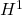and a function of bounded mean oscillation can be written, in the sense of distributions, as a sum of an integrable function and an element in an appropriate Hardy-Orlicz space. Moreover, they showed that the decomposition operators can be taken to be bilinear.

In this talk we present a dyadic analogue of the result of Bonami, Grellier, and Ky and we show how their theorem can be recovered from its dyadic counterparts. Related topics in one and several parameters will also be discussed.

This is joint work Sandra Pott, Salvador Rodríguez-López, and Alan Sola.

Friday, 4th February 2022

### Michael Tang (University of Edinburgh)

Abstract:

The multijoint problem is a discrete analogue to the multilinear Kakeya problem, and was solved by Zhang in 2016. More recently in 2020, Tidor, Yu and Zhao solved higher-dimensional generalisations of the multijoint problem. The multijoint and multilinear Kakeya problems can both be described by geometric multilinear inequalities for which there is a theory of duality, as established by Carbery, Hanninen and Valdimarsson. While the dual multilinear Kakeya problem is well understood, until now, the dual multijoint problem was not, despite the multijoint problem being solved by Zhang in 2016.

In this talk we formulate the multijoint problem as the boundedness of a multilinear operator and present a discrete analogue of a theorem due to Bourgain and Guth.

Friday, 11th February 2022

### Christoph Kehle (Insitute for Advanced Study)

Abstract:

The statement that general relativity is a deterministic theory finds its mathematical formulation in the celebrated ‘Strong Cosmic Censorship Conjecture’ due to Roger Penrose. I will present my recent results on the linear analog of this conjecture in the case of negative cosmological constant. It turns out that this is intimately tied to Diophantine properties of a suitable ratio of mass and angular momentum of the black hole and that the validity of the conjecture depends in an unexpected way on the notion of genericity imposed.

Friday, 25th February 2022

### João Pedro Ramos (ETH Zürich)

Abstract:

The celebrated isoperimetric inequality states that, for a measurable set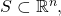the inequality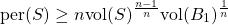holds, where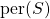denotes the perimeter (or surface area) of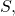and equality holds if and only if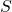is an euclidean ball. This result has many applications throughout analysis, but an interesting feature is that it can be obtained as a corollary of a more general inequality, the Brunn–Minkowski theorem: if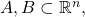define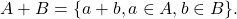Then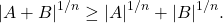Here, equality holds if and only if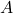and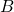are homothetic and convex. A question pertaining to both these results, that aims to exploit deeper features of the geometry behind them, is that of stability: ifis close to being optimal for the isoperimetric inequality, can we say thatis close to being a ball? Analogously, if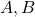are close to being optimal for Brunn–Minkowski, can we say they are close to being compact and convex?

These questions, as stand, have been answered only in very recent efforts by several mathematicians. In this talk, we shall outline these results, with focus on the following new result, obtained jointly with A. Figalli and K. Boroczky. If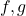are two non-negative measurable functions on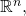and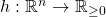is measurable such that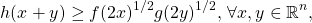then the Prekopa–Leindler inequality asserts that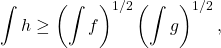where equality holds if and only if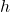is log-concave, andare `homothetic’ to, in a suitable sense. We prove that, if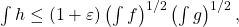then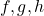are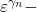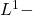close to being optimal. We will discuss the general idea for the proof and, time-allowing, discuss on a conjectured sharper version.

Friday, 4th March 2022

### Priscila Leal da Silva (Loughborough)

Abstract:

Since the discovery of the Camassa-Holm equation as an integrable peakon-equation, intense research has been dedicated to its generalisations of it and to the study of their solutions. Of particular interest in this talk is the rotation-Camassa-Holm equation (rCH), a nonlocally evolutive equation that takes into consideration the Coriolis force, which is typically a manifestation of rotation when Newton’s laws are applied to model physical phenomena on Earth’s surface. In this talk we present conditions for the existence of traveling wave solutions and show that, given an initial data in Sobolev spaces, uniqueness of local solutions is achieved. Moreover, assuming a strong condition on the McKean quantity, the solutions can be extended globally. Extensions of these results are presented, as well as further conjectures.

Friday, 11th March 2022

### Julian Braun (Heriot–Watt)

Abstract:

Lattice defects in crystalline materials (for example, a screw dislocations or an interstitial) create long-range elastic fields. In this presentation I will show how to rigorously derive a far-field expansion up to arbitrary high order. The expansion is computable and leaves only a fast decaying remainder describing the defect core structure. As an application I will also show how to use this expansion for high accuracy defect computations.

Friday, 18th March 2022

### Louise Gassot (ICERM  / University of Basel)

Abstract:

We discuss the zero-dispersion limit for the Benjamin-Ono equation on the torus given a single well initial data. We prove that there exist approximate initial data converging to the initial data, such that the corresponding solutions admit a weak limit as the dispersion parameter tends to zero. The weak limit is expressed in terms of the multivalued solution of the inviscid Burgers equation obtained by the method of characteristics. We construct our approximation by using the Birkhoff coordinates of the initial data, introduced by Gérard, Kappeler and Topalov.

Friday, 1st April 2022

### Annalisa Massaccesi (Università di Padova)

Abstract:

Abstract: In this seminar I will review the theory of flat G-chains, as they were introduced by H. W. Fleming in 1966, and currents with coefficients in groups. One of the most recent developments of the theory concerns its application to the Steiner tree problem and other minimal network problems which are related with a Eulerian formulation of the branched optimal transport. Starting from a 2016 paper by A. Marchese and myself, I will show how these problems and their variants are equivalent to a mass-minimization problem in the framework of currents with coefficients in a (suitably chosen) normed group.

Friday, 29 April 2022

### Ian Wood (University of Kent)

Abstract:
We determine and classify the spectrum of a non-selfadjoint operator pencil generated by the time-harmonic Maxwell problem with a nonlinear dependence on the frequency. More specifically, we consider one- and two-dimensional reductions for the case of two homogeneous materials joined at a planar interface. The dependence on the spectral parameter, i.e. the frequency, is in the dielectric function and we make no assumptions on its form. In order to allow also for non-conservative media, the dielectric function is allowed to be complex, yielding a non-selfadjoint problem. This is joint work with Malcolm Brown (Cardiff), Tomas Dohnal (Halle) and Michael Plum (Karlsruhe).

Friday, 13 May 2022

### Boris Thibert (Universite Grenoble Alpes)

Abstract:
Generated Jacobian Equations have been introduced in 2014 by Trudinger as a generalization of Monge-Ampère equations arising in optimal transport. They were originally motivated by inverse problems arising in non-imaging optics, where the goal is to design optical components, such as mirrors or lenses, that transfer a given source light to a prescribed target light.In this talk, I will present a Newton algorithm for solving Generated Jacobian equations in the semi-discrete setting, meaning that one of the measures involved in the problem is finitely supported and the other one is absolutely continuous. Then I will apply it to the design of different kinds of mirrors or lenses that allow to transfer any punctual or collimated source to any target. This work is in collaboration with Anatole Gallouet and Quentin Mérigot.

# Previous semesters

## Autumn 2021

Friday, 1st October 2021

### Matias Ruiz (University of Edinburgh)

Abstract:

In the last couple of decades, the field of nanophotonics, i.e. the study of light-matter interactions at the nanoscale, has gained enormous importance in different disciplines of science and engineering. A large portion of this field concerns the study of electromagnetic resonances in nanoparticles which are, in turn, the building blocks of many applications in energy, healthcare, material science, to name a few.

The description of these resonances relies on interesting math problems with strong connections to PDE spectral problems, both self-adjoint and non-self-adjoint. As such, there is a growing interest from the math world in tackling some of the mathematical issues arising in nanophotonics. For instance, a canonical problem is the Laplace transmission problem showing a sign-change in the PDE coefficients, whose solutions can be understood in terms of the spectral properties of the Neumann-Poincare operator (and/or the so-called plasmonic eigenvalue problem).

In this applied analysis talk I will introduce some of the mathematically interesting problems arising in nanophotonics and discuss recent analytical results in the analysis of resonances in metallic nanoparticles (also known as plasmonic resonances), which include the use of layer potential techniques and asymptotic analysis.

Friday, 8th October 2021

### Jean-Claude Cuenin (Loughborough University)

Abstract:

I will give a survey of how methods from harmonic analysis, particularly those related to Fourier restriction theory, can be used in spectral and scattering theory. Applications include eigenvalue estimates for Schrödinger operators with complex potentials and almost sure scattering for random lattice Schrödinger operators in 3d with slowly decaying potential.

Friday, 15th October 2021

### Simon Myerson (University of Warwick)

Abstract:

Given the Laplace(-Beltrami) operator on a Riemannian manifold, consider the spectral projector on (generalized) eigenfunctions whose eigenvalue lies in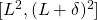– where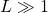and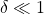. We ask the question of optimal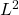to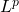bounds for this operator. I will present new results in this direction for the Euclidean torus (joint with Pierre Germain).

Friday, 22nd October 2021

### Guido De Philippis (CIMS)

Abstract:

Michael Simon inequality is a fundamental tool in  geometric analysis and geometric measure theory.  Its extension to anisotropic integrands will allow to extend to anisotropic integrands a series of results which are currently known only for the area functional.

In this talk I will present an anistropic  version of the Michael-Simon inequality, for for two-dimensional varifolds in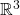, provided that the integrand is close to the area in the C1-topology. The proof is deeply inspired by posthumous notes by Almgren, devoted to the same result. Although our arguments overlap with Almgren’s, some parts are greatly simplified and rely on a nonlinear version of the planar multilinear Kakeya inequality.

Friday, 29th October 2021

### Myrto Maria Galanopoulou (Heriot-Watt University)

Abstract:

In this talk, I will present the findings of my PhD Thesis. I will examine the system of thermoelasticity endowed with polyconvex energy. I will show that we can embed the equations of polyconvex thermoviscoelasticity into an augmented, symmetrizable, hyperbolic system which possesses a convex entropy. This allows to prove many important stability results, such as convergence from the viscous problem to smooth solutions of the system of adiabatic thermoelasticity in the zero-viscosity limit. In addition, I will present a weak-strong uniqueness result in the class of entropy weak solutions and in a suitable class of measure-valued solutions, defined by means of generalized Young measures. Also, we will discuss a variational scheme for isentropic processes of adiabatic polyconvex thermoelasticity: I establish existence of minimizers which converge to a measure-valued solution that dissipates the total energy, while the scheme converges when the limiting solution is smooth.

Friday, 5th November 2021

### Gigliola Staffilani (MIT)

Abstract:

In this talk I will outline some results on the study of transfer of energy for solutions to the periodic 2D (torus domain) cubic defocusing nonlinear Schrodinger equation. In particular I will focus on the differences of the dynamics of solutions in the rational versus irrational torus. Some numerical experiments will also be presented.

(The most recent work presented is in collaboration with A. Hrabski, Y. Pan and B. Wilson.)

Friday, 19th November 2021

### Laure Saint-Raymond (IHES)

Abstract:

The dynamics of a hard sphere gas is described in the low density limit by the Boltzmann equation, at least for short times. The classical strategy  of proof, due to Lanford, fails for longer times, even close to equilibrium.In this talk, I will present a recent work with T. Bodineau, I. Gallagher and S. Simonella, where we  have  designed a new method, combining a duality and  a pruning arguments, which allows  to characterize the fluctuations around equilibrium globally in time.

Friday, 26th November 2021

### Jim Wright (University of Edinburgh)

Abstract:

In 1988 Bourgain gave a quantitative count for the number of progressions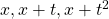in dense sets on the real line. Recently this was extended to general 3-term polynomial progressions by  X. Chen, J. Guo and X. Li. In this talk we extend these results to arbitrarily long polynomial progressions. Our methods are robust enough
to give quantitative counts for long polynomial progressions in any locally compact topological field with nontrivial topology. This is joint work with B. Krause, M. Mirek and S. Peluse.

Friday, 10th December 2021

### Jan Sbierski (University of Edinburgh)

Abstract:

The strong cosmic censorship conjecture roughly states that Einstein’s theory of general relativity is generically a deterministic theory. I will start out this talk by giving a non-technical introduction to this conjecture restricted to rotating black holes. After motivating the mathematical formulation of the strong cosmic censorship conjecture I will proceed to outline the current state of it and then conclude by presenting forthcoming work of mine showing the linear instability of the Cauchy horizon inside rotating black holes.

## Spring 2021

Friday, 22nd January 2021

### Federico Luigi Dipasquale (Università di Verona)

Abstract:

I will talk about recent results on the topology of minimizers in the Landaude Gennes (LdG) theory of nematic liquid crystals which shed light on the socalled “biaxial torus minimizers”. According to many numerical studies, these are the minimizers of the most studied LdG energy in very relevant physical conditions. Such minimizers are axially symmetric and characterized by remarkable topological properties (where the topology is to be sought in the level set of an appropriate indicator function, the signed biaxiality). However, they eluded precise mathematical description for years. In this talk, I will introduce some new key ideas which allow to study the topology of minimizers (but also of some classes of more general configurations). I will try to explain how to implement them and how this leads in a natural way to the definition of an appropriate asymptotic regime in which, in fact, topological structures are found in the biaxiality sets of minimizers. The topological results match qualitatively many features expected from the numerical simulations. We will also see that, restricting to axially symmetric configurations, we have still better accordance with simulations and much more can be said, although several interesting problems remain open. Joint work with V. Millot (Paris XIII) and A. Pisante (Sapienza).

Friday, 29th January 2021

### Franz Gmeineder (Universität Bonn)

Abstract:

By Morrey’s foundational work, quasiconvexity displays a key notion in the vectorial Calculus of Variations. A suitable generalisation that keeps track of more elaborate differential conditions is given by Fonseca & Müller’s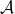-quasiconvexity. With the topic having faced numerous contributions as to lower semicontinuity, in this talk I give an overview of recent results for such problems with focus on the underlying function spaces and the (partial) regularity of minima. The talk is partially based on joint work with Sergio Conti (Bonn), Jan Kristensen (Oxford) and Lars Diening (Bielefeld).

Friday, 5th February 2021

### Eugenia Malinnikova (Stanford)

Abstract:

We consider a real-valued function on the plane for which the absolute value of the Laplacian is bounded by the absolute value of the function at each point. In other words, we look at solutions of the stationary Schrödinger equation with a bounded potential. The question discussed in the talk is how fast such function may decay at infinity. We give the answer in dimension two, in higher dimensions the corresponding problem is open. The talk is based on the joint work with A. Logunov, N. Nadirashvili, and F. Nazarov.

Friday, 12th February 2021

### Stefan Krömer (Czech Academy of Sciences)

Abstract:

Globally invertible Sobolev maps are of particular interest in models for the elastic deformation of solids, because invertibility corresponds to non-interpenetration of matter. By a result of John Ball (1981), a locally orientation preserving Sobolev map is almost everywhere globally invertible provided that its boundary values admit a homeomorphic extension. We will see that the conclusions of Ball’s theorem and related results can be reached while completely avoiding the (hard) problem of homeomorphic extension. The proof heavily relies on Brouwer’s topological degree. Among other things, the result can be applied to justify more efficient numerical approximation respecting global invertibility constraints.

Friday, 19th February 2021

### Mihalis Dafermos (Cambridge/Princeton)

Friday, 26th February 2021

### Hyunju Kwon (IAS)

Abstract:

The Euler equations describe the behavior of ideal fluids. It is well-known that smooth (spatially periodic) Euler flows conserve total kinetic energy in time. In the theory of turbulence, on the other hand, a famous conjecture of Lars Onsager asserts that kinetic energy conservation may fail when an Euler flow belongs to the Hölder spaces with Hölder exponent less than 1/3, which was proved recently by Isett. In light of these developments, I’ll discuss a stronger version of the Onsager conjecture: the existence of Hölder continuous Euler flows which locally dissipate kinetic energy.

Friday, 5th March 2021

### Jill Pipher (Brown)

Abstract:

Formulating and solving boundary value problems for divergence form real elliptic equations has been an active and productive area of research ever since the foundational work of De Giorgi – Nash – Moser established H\”older continuity of solutions when the operator coefficients are merely bounded and measurable. The solutions to such real-valued equations share some important properties with harmonic functions: maximum principles, Harnack principles, and estimates up to the boundary that enable one to solve Dirichlet problems in the classical sense of nontangential convergence. Weak solutions of complex elliptic equations and elliptic systems do not necessarily share these good properties of continuity or maximum principles. In joint work with M. Dindos, we introduced in 2017 a structural condition (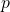-ellipticity) on divergence form complex elliptic equations that was inspired by a condition related tocontractivity due to Cialdea and Maz’ya. The-ellipticity condition was also simultaneously discovered by Carbonaro-Dragicevic, who used it to prove a bilinear embedding result. Subsequently, the condition has proven useful in the study of well-posedness of a degenerate elliptic operator associated with domains with lower-dimensional boundary. In this talk we discuss-ellipticity for complex divergence form equations, and then describe recent work, joint with J. Li and M. Dindos, extending this condition to elliptic systems. In particular, we give applications to solvability of the Dirichlet problem for the Lame systems.

Friday, 12th March 2021

### Ilaria Fragalé (Politecnico di Milano)

Abstract:

We discuss the rigidity of measurable subsets in the Euclidean space such that the Lebesgue measure of their intersection with a ball of radius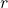, centred at any point in the essential boundary, is constant. Based on a joint work with Dorin Bucur.

Friday, 19th March 2021

### Matthew Hyde (University of Edinburgh)

Abstract:

In the nineties, Peter Jones proved the Analyst’s Travelling Salesman Theorem (TST), which gives a necessary and sufficient condition for when a set in Euclidean space can be contained in a curve of finite length. This condition is stated in terms of beta-numbers, which give a measure of how flat a set is at each location and scale. Several higher dimensional analogues of the TST have been proven since. These, along with Jones’ original result, have found many applications in fields such as complex analysis, harmonic analysis, and harmonic measure. Unlike Jones’ TST, these higher dimensional variants require additional assumption on the set. In this talk we will discuss these results in more detail and introduce a higher dimensional TST that holds for every set in Euclidean space.

Friday, 26th March 2021

### Joris Roos (University of Massachusetts Lowell & University of Edinburgh)

Abstract:

Stein and Wainger introduced an interesting class of maximal oscillatory integral operators related to Carleson’s theorem. The talk will be about joint work with Ben Krause on discrete analogues of some of these operators. These discrete analogues feature a number of substantial difficulties that are absent in the real-variable setting and involve themes from number theory and analysis.